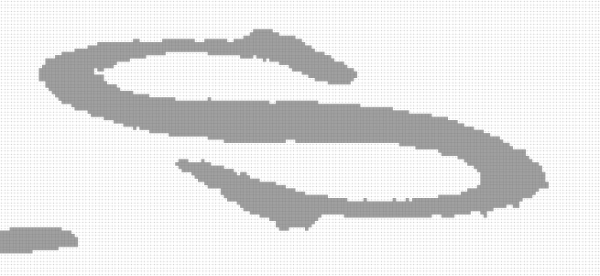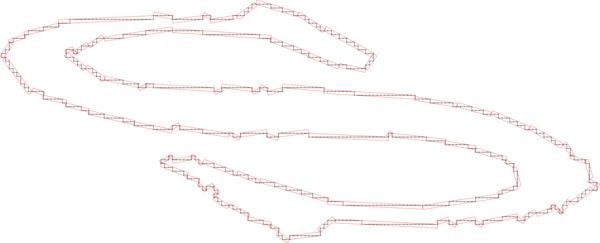DGtal  1.1.0
Tutorial "Image -> Region -> Grid curve -> Length estimation"

Author(s) of this documentation:
Tristan Roussillon

In this example, we show how to retrieve the contour of a connected subset of an image and estimate its perimeter.

# Extracting a digital set from an image

Let us assume that the following image has been loaded into an image object:S gray level image

First of all, we implicitely extract a digital set from an image, using a simple threshold on gray level values:

//predicate from the image
Binarizer b(1, 135);
PointFunctorPredicate<Image,Binarizer> predicate(image, b);
Note
The explicit construction of the digital set is not necessary.
Since the domain of the image is known (methods lowerBound() and upperBound()), it is indeed enough to have a predicate that indicates, for each point of the domain, whether it belongs to the digital set or not.

This predicate returns true for each dark pixel of the following image:S binary image

# Extracting the contours of a digital set

The extraction of the contour is performed in a cellular space (with 0-, 1-, and 2-cells), given an adjacency (0-, 1-, 2-adjacency). See Cellular grid space and topology, unoriented and oriented cells, incidence for the basic concepts of cellular topology.

Z2i::KSpace ks; //Khalimsky space
ks.init( image.domain().lowerBound(), image.domain().upperBound(), true );

Then, the contour of each connected component can be retrieved as follows:

//extraction of all the contours
std::vector< std::vector< Z2i::SCell > > contours;
::extractAll2DSCellContours( contours, ks, sAdj, predicate );

# Grid curve instantiation

The GridCurve object is merely built from one retrieved contour, which is stored as a vector of signed 1-cells:

//init grid curve from the first retrieved contour
c.initFromSCellsVector( contours.at(1) );

# Length estimation

Now, we want to get a perimeter estimation of the grid curve c. Several length estimators have been implemented in DGtal, but we are using in what follows the one based on a greedy segmentation of the curve into digital straight segments (DSS).

Since the DSS recognition algoritm deals with digital points, we get the points range of the grid curve c.

//range of points
Range r = c.getPointsRange();

The DSS segmentation looks like this:DSS segmentation

Then, we initialize the DSS length estimator from the points range and get the estimated length.

//length estimation based on a DSS segmentation
DSSLengthEstimator< Range::ConstCirculator > DSSlength;
trace.info() << "Length: " << DSSlength.eval(r.c(), r.c(), 1.) << std::endl;

You should see in the standard output:

Length: 723.238

# Required includes

You should include these common files:

#include "DGtal/base/Common.h"
#include "DGtal/helpers/StdDefs.h"
#include "ConfigExamples.h"

#include "DGtal/base/BasicFunctors.h"
#include "DGtal/kernel/BasicPointPredicates.h"
#include "DGtal/images/ImageContainerBySTLVector.h"

For extracting the contours of the digital set:

#include "DGtal/topology/helpers/Surfaces.h"

And finally, for estimating the length of the retrieved contours, you should include this:

#include "DGtal/geometry/curves/estimation/DSSLengthEstimator.h"
DGtal::KhalimskySpaceND::init
bool init(const Point &lower, const Point &upper, bool isClosed)
Specifies the upper and lower bounds for the maximal cells in this space.
DGtal::trace
Trace trace
Definition: Common.h:150
DGtal::Trace::info
std::ostream & info()
DGtal::SimpleRandomAccessRangeFromPoint
Aim: model of CBidirectionalRangeFromPoint that adapts any range of elements bounded by two iterators...
Definition: SimpleRandomAccessRangeFromPoint.h:74
DGtal::Z2i::KSpace
KhalimskySpaceND< 2, Integer > KSpace
Definition: StdDefs.h:77
DGtal::Z2i::Curve
GridCurve< K2 > Curve
Definition: StdDefs.h:116
Range
Image::Range Range
Definition: testSimpleRandomAccessRangeFromPoint.cpp:46
DGtal::Surfaces::extractAll2DSCellContours
static void extractAll2DSCellContours(std::vector< std::vector< SCell > > &aVectSCellContour2D, const KSpace &aKSpace, const SurfelAdjacency< KSpace::dimension > &aSurfelAdj, const PointPredicate &pp)
DGtal::GridCurve::PointsRange
ConstRangeAdapter< typename Storage::const_iterator, functors::SCellToPoint< KSpace >, Point > PointsRange
Definition: GridCurve.h:421
DGtal::functors::IntervalThresholder
Aim: A small functor with an operator () that compares one value to an interval.
Definition: BasicFunctors.h:701